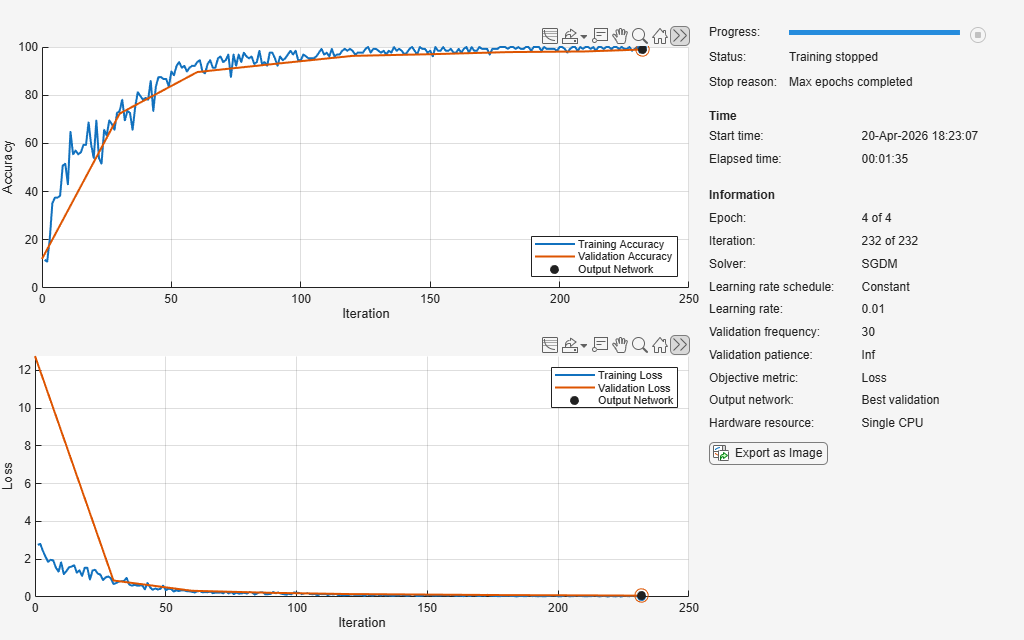# Create Simple Image Classification Network

This example shows how to create and train a simple convolutional neural network for deep learning classification. Convolutional neural networks are essential tools for deep learning and are especially suited for image recognition.

The example demonstrates how to:

• Define the network architecture.

• Specify training options.

• Train the network.

• Predict the labels of new data and calculate the classification accuracy.

Load the digit sample data as an image datastore. The `imageDatastore` function automatically labels the images based on folder names.

```digitDatasetPath = fullfile(matlabroot,'toolbox','nnet','nndemos', ... 'nndatasets','DigitDataset'); imds = imageDatastore(digitDatasetPath, ... 'IncludeSubfolders',true, ... 'LabelSource','foldernames');```

Divide the data into training and validation data sets, so that each category in the training set contains 750 images, and the validation set contains the remaining images from each label. `splitEachLabel` splits the image datastore into two new datastores for training and validation.

```numTrainFiles = 750; [imdsTrain,imdsValidation] = splitEachLabel(imds,numTrainFiles,'randomize');```

### Define Network Architecture

Define the convolutional neural network architecture. Specify the size of the images in the input layer of the network and the number of classes in the fully connected layer before the classification layer. Each image is 28-by-28-by-1 pixels and there are 10 classes.

```inputSize = [28 28 1]; numClasses = 10; layers = [ imageInputLayer(inputSize) convolution2dLayer(5,20) batchNormalizationLayer reluLayer fullyConnectedLayer(numClasses) softmaxLayer classificationLayer];```

### Train Network

Specify the training options and train the network.

By default, `trainNetwork` uses a GPU if one is available (requires Parallel Computing Toolbox™ and a CUDA® enabled GPU with compute capability 3.0 or higher). Otherwise, it uses a CPU. You can also specify the execution environment by using the `'ExecutionEnvironment'` name-value pair argument of `trainingOptions`.

```options = trainingOptions('sgdm', ... 'MaxEpochs',4, ... 'ValidationData',imdsValidation, ... 'ValidationFrequency',30, ... 'Verbose',false, ... 'Plots','training-progress'); net = trainNetwork(imdsTrain,layers,options);```### Test Network

Classify the validation data and calculate the classification accuracy.

```YPred = classify(net,imdsValidation); YValidation = imdsValidation.Labels; accuracy = mean(YPred == YValidation)```
```accuracy = 0.9892 ```

For next steps in deep learning, you can try using pretrained network for other tasks. Solve new classification problems on your image data with transfer learning or feature extraction. For examples, see Start Deep Learning Faster Using Transfer Learning and Train Classifiers Using Features Extracted from Pretrained Networks. To learn more about pretrained networks, see Pretrained Deep Neural Networks.